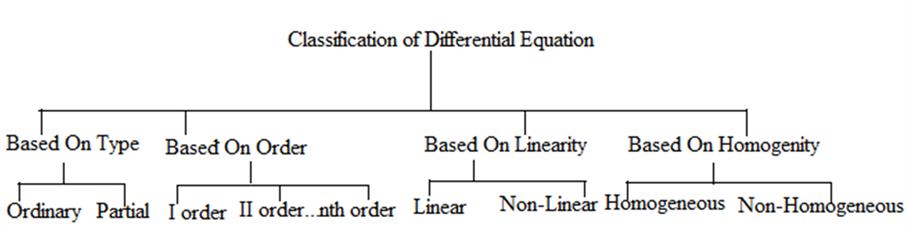# Differential Equation And Its Types

Before knowing about Differential Equation and its types, let us know what a differential equation is. An equation with one or more terms that involve derivatives of the dependent variable with respect to an independent variable is known as Differential Equation.

An equation with one or more terms that involve derivatives of the dependent variable with respect to an independent variable is known as a differential equation.

In simple words, a differential equation consists of derivatives, which could either be ordinary derivatives or partial derivatives.

Example:

$$\begin{array}{l} \frac {d^2y}{dx^2} + \left( \frac {dy}{dx} \right)^2 \end{array}$$

$$\begin{array}{l} \frac {d^2y}{dt^2} + \frac {dy}{dt} = 5 sin t \end{array}$$

## Classification of Differential Equation

The below given tree is the general classification of differential equation.Now let us learn all the types with detailed explanations.

## Differential Equations- Based on Type

There are two types of differential equation:

1. Ordinary Differential Equation
2. Partial Differential Equation

### Ordinary Differential Equation

Ordinary differential equation which depends on a single independent variable.

Example:

$$\begin{array}{l} \frac {dy}{dx} + 5x = 5y \end{array}$$

(b)   Partial Differential Equation

It involves partial derivatives.

$$\begin{array}{l} \frac {\partial y}{\partial x} + \frac {\partial y}{\partial t} = x^3 – t^3 \end{array}$$
……….(i)

$$\begin{array}{l} \frac {\partial^2 y}{\partial x^2} – c^2 \frac {\partial^2 y}{\partial t^2} = x0 \end{array}$$
……………..(ii)

## Differential Equations- Based on Order

The order of the highest differential coefficient (derivative) involved in the differential equation is known as the order of the differential equation.

For Example:-

$$\begin{array}{l} \frac {d^3y}{dx^2} + 5 \frac {dy}{dx} + y = \sqrt{x} \end{array}$$

Here, the order = 3 as the order of the highest derivative involved is 3.

For derivatives the use of single quote notation is preferred which is

$$\begin{array}{l} y’ = \frac {dy}{dx} \end{array}$$
.

$$\begin{array}{l} y” = \frac {d^2y}{dx^2} \end{array}$$

$$\begin{array}{l} y”’ = \frac {d^3y}{dx^3} \end{array}$$

and so on

For the higher order derivatives it would become cumbersome to use multiple quotes so for these derivatives we prefer using the notation yn  for the nth order derivative

$$\begin{array}{l} \frac {d^ny}{dx^n} \end{array}$$
.

Consider the following examples:-

(i) y” + 5y’ – 6y =

$$\begin{array}{l} x^2 \end{array}$$
+ 3x

(ii) x’ = -x + 16

(iii) x”’ + 2x’ = 0

The equation (i) is a second order differential equation as the order of the highest differential co-efficient is 2.

Similarly, the example is a first-order differential equation as the highest derivative is of order 1.

The example is a third-order Differential Equation.

## Differential Equations – Based on Linearity

By linearity, it means that the variable appearing in the equation is raised to the power of one. The graph of linear functions is generally a straight line. For example: (3x + 5) is Linear but (x3 + 4x2) is  non-linear.

### Linear Differential Equation

If all the dependent variables and its entire derivatives occur linearly in a given equation, then it represents a linear differential equation.

### Non-Linear Differential Equations

Any differential equation with non-linear terms is known as a non-linear differential equation.

Consider the following examples for illustration:

Example 1:

$$\begin{array}{l} \frac {dy}{dx} + xy = 5x \end{array}$$
……… (i)

$$\begin{array}{l} \frac {d^2y}{dx^2} – ln y = 10 \end{array}$$
………. (ii)

Example 1:

$$\begin{array}{l} \frac {dy}{dx} + xy = 5x \end{array}$$

It is a linear differential equation as

$$\begin{array}{l} \frac {dy}{dx} \end{array}$$
and  both are linear.

Example 2:

$$\begin{array}{l} \frac {d^2 y}{dx^2} – ln \space y =10 \end{array}$$

In y is not linear. Hence, this equation is non-linear.

## Differential Equations – Based on Homogeneity

Consider the following functions:

$$\begin{array}{l} f_1(x, y) = y^3 + \frac 23 xy^2 \end{array}$$

$$\begin{array}{l} f_2(x, y) = x^3 ÷ y^2 x \end{array}$$

$$\begin{array}{l} f_3(x, y) = tan x + sec y \end{array}$$

If we replace x and y by αx  and αy respectively, where α is any non-zero constant, we get;

$$\begin{array}{l} f_1(x,y) = (\alpha y )^3 + \frac 23 (\alpha x) (\alpha y)^2 = \alpha^3 ( y^3 + \frac 23 xy ) = \alpha^3 f_1 (x,y) \end{array}$$

$$\begin{array}{l} f_2 (x,y) = \frac {(\alpha x)^3}{(\alpha y)^3 (\alpha x)} = \frac {x^3}{xy^2} = \alpha^\circ f_2 (x,y) \end{array}$$

$$\begin{array}{l} f_3 (x,y) = tan (\alpha x) + sex (\alpha y) \end{array}$$

We observe that,

$$\begin{array}{l} f_1,f_2 \end{array}$$
can be written in the form
$$\begin{array}{l} f(αx, αy) = α^n f(x, y) \end{array}$$
but this is not applicable to
$$\begin{array}{l} f_3 (x, y) \end{array}$$
Therefore, if a function satisfies the condition that
$$\begin{array}{l} f (αx, xy) = α^n f(x, y) \end{array}$$
for a non-zero constant α, it is known as homogeneous equation of degree n.

The linear differential equation of the form,

$$\begin{array}{l} fn (x) y^n + …….+ f_1 (x)y’ + f0(x) y = g(x) \end{array}$$
represents a homogeneous differential equation if the R.H.S is zero i.e.,g(x) = 0 , Elseit represents non-homogeneous differential equation if g(x)
$$\begin{array}{l} \ne \end{array}$$
0.